## Example Questions

### Example Question #1 : How To Find An Angle In A Trapezoid

Find the measure of anglein the isosceles trapezoid pictured below.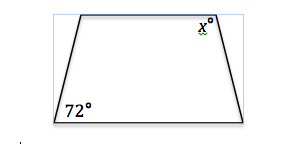Possible Answers: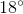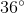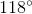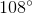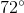Correct answer:Explanation:

The sum of the angles in any quadrilateral is 360°, and the properties of an isosceles trapezoid dictate that the sets of angles adjoined by parallel lines (in this case, the bottom set and top set of angles) are equal. Subtracting 2(72°) from 360° gives the sum of the two top angles, and dividing the resulting 216° by 2 yields the measurement of x, which is 108°.

### Example Question #2 : How To Find An Angle In A Trapezoid

Trapezoidis an isosceles trapezoid with angle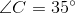. If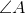and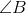are paired, what is the measure of?

Possible Answers: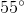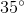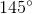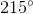Correct answer:Explanation:

As a rule, adjacent (non-paired) angles in a trapezoid are supplementary. Thus, we know that if, then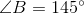. Since we are told thatandare paired and trapezoidis isosceles,must also equal.

### Example Question #3 : How To Find An Angle In A Trapezoid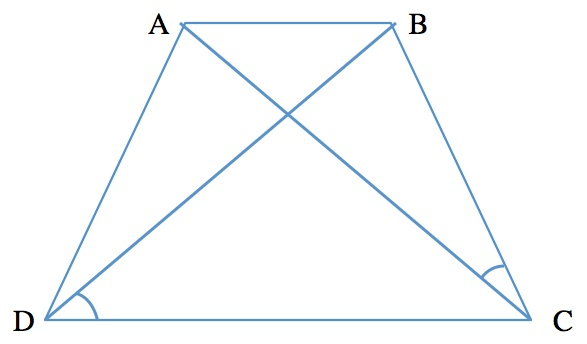In the isosceles trapezoid above,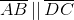.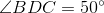and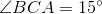.

In degrees, what is the measure of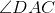?

Possible Answers: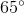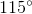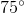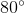Correct answer:Explanation:

To find the measure of angle DAC, we must know that the interior angles of all triangles sum up to 180 degrees. Also, as this is an isosceles trapezoid,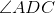and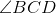are equal to each other. The two diagonals within the trapezoid bisect anglesandat the same angle.

Thus,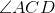must also be equal to 50 degrees.

Thus,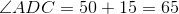.

Now that we know two angles out of the three in the triangle on the left, we can subtract them from 180 degrees to find: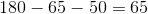### Example Question #4 : How To Find An Angle In A Trapezoid

Given the following isosceles triangle: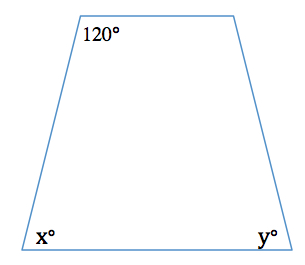In degrees, find the measure of the sum of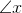and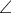in the figure above.

Possible Answers: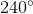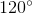Correct answer:Explanation:

All quadrilaterals' interior angles sum to 360°. In isosceles trapezoids, the two top angles are equal to each other.

Similarly, the two bottom angles are equal to each other as well.

Therefore, to find the sum of the two bottom angles, we subtract the measures of the top two angles from 360: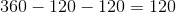### All ACT Math Resources